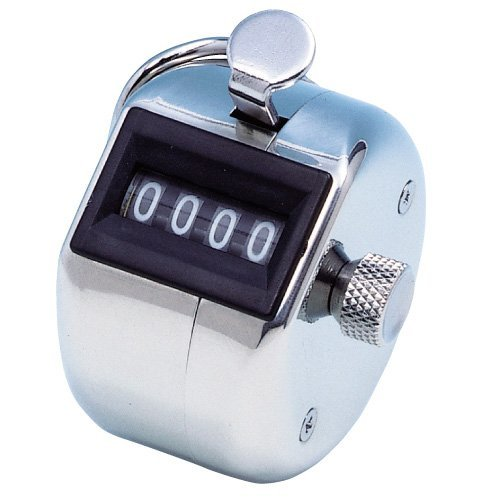# How millis() resets itself to 0

Looking at the documentation for the `millis()` function , it says:

Returns the number of milliseconds since the Arduino board began running the current program. This number will overflow (go back to zero), after approximately 50 days.

How's this possible? Is Arduino detecting when `millis()` overflow then resets the value to 0? If so, how does it do it? I just want to know what exactly is going on under the hood with the variable that is being returned by the `millis()` function.

It is not an exclusive property of `millis()`. Any counter with a limited number of digits eventually returns to zero. For example, a 4 digit tally counter returns to zeros after `9999`.
Under the hood, the variable for `millis()` is of type `unsigned long`, which is 32 bits on the Arduino. In this case, the 32 bits (binary 0's and 1's) are similar to the digits (0 thru 9) on the tally counter.
The maximum is `11111111 11111111 11111111 11111111`. Once there, `millis()` returns to zero when you add `1`. The maximum (32 "ones") is `2^32 - 1`, which equals `4294967295` in the decimal number system. The maximum value for the counter is, therefore, 4,294,967,295 milliseconds. Converted to days you get approximately 49.71 days.